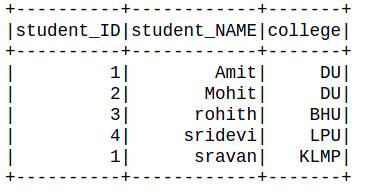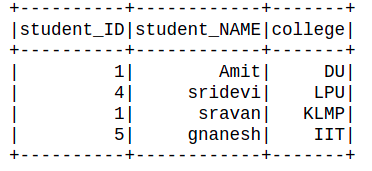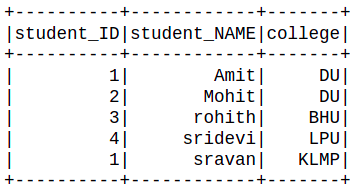# Delete rows in PySpark dataframe based on multiple conditions

In this article, we are going to see how to delete rows in PySpark dataframe based on multiple conditions.

### Method 1: Using Logical expression

Here we are going to use the logical expression to filter the row. Filter() function is used to filter the rows from RDD/DataFrame based on the given condition or SQL expression.

Syntax: filter( condition)

Parameters:

• Condition: Logical condition or SQL expression

Example 1:

## Python3

 `# importing module ` `import` `pyspark ` ` `  `# importing sparksession from pyspark.sql ` `# module ` `from` `pyspark.sql ``import` `SparkSession ` ` `  `# spark library import ` `import` `pyspark.sql.functions ` ` `  `# creating sparksession and giving an app name ` `spark ``=` `SparkSession.builder.appName(``'sparkdf'``).getOrCreate() ` ` `  `# list  of students  data ` `data ``=` `[[``"1"``, ``"Amit"``, ``" DU"``], ` `        ``[``"2"``, ``"Mohit"``, ``"DU"``], ` `        ``[``"3"``, ``"rohith"``, ``"BHU"``], ` `        ``[``"4"``, ``"sridevi"``, ``"LPU"``], ` `        ``[``"1"``, ``"sravan"``, ``"KLMP"``], ` `        ``[``"5"``, ``"gnanesh"``, ``"IIT"``]] ` ` `  `# specify column names ` `columns ``=` `[``'student_ID'``, ``'student_NAME'``, ``'college'``] ` ` `  `# creating a dataframe from the lists of data ` `dataframe ``=` `spark.createDataFrame(data, columns) ` ` `  `dataframe ``=` `dataframe.``filter``(dataframe.college !``=` `"IIT"``) ` ` `  `dataframe.show() `

Output:Example 2:

## Python3

 `# importing module ` `import` `pyspark ` ` `  `# importing sparksession from pyspark.sql ` `# module ` `from` `pyspark.sql ``import` `SparkSession ` ` `  `# spark library import ` `import` `pyspark.sql.functions ` ` `  `# creating sparksession and giving an app name ` `spark ``=` `SparkSession.builder.appName(``'sparkdf'``).getOrCreate() ` ` `  `# list  of students  data ` `data ``=` `[[``"1"``, ``"Amit"``, ``" DU"``], ` `        ``[``"2"``, ``"Mohit"``, ``"DU"``], ` `        ``[``"3"``, ``"rohith"``, ``"BHU"``], ` `        ``[``"4"``, ``"sridevi"``, ``"LPU"``], ` `        ``[``"1"``, ``"sravan"``, ``"KLMP"``], ` `        ``[``"5"``, ``"gnanesh"``, ``"IIT"``]] ` ` `  `# specify column names ` `columns ``=` `[``'student_ID'``, ``'student_NAME'``, ``'college'``] ` ` `  `# creating a dataframe from the lists of data ` `dataframe ``=` `spark.createDataFrame(data, columns) ` ` `  `dataframe ``=` `dataframe.``filter``( ` `    ``((dataframe.college !``=` `"DU"``) ` `     ``& (dataframe.student_ID !``=` `"3"``)) ` `) ` ` `  `dataframe.show() `

Output:### Method 2: Using when() method

It evaluates a list of conditions and returns a single value. Thus passing the condition and its required values will get the job done.

Syntax: When( Condition, Value)

Parameters:

• Condition: Boolean or columns expression.
• Value: Literal Value

Example:

## Python3

 `# importing module ` `import` `pyspark ` ` `  `# importing sparksession from pyspark.sql  ` `# module ` `from` `pyspark.sql ``import` `SparkSession ` ` `  `# spark library import ` `import` `pyspark.sql.functions ` ` `  `# spark library import ` `from` `pyspark.sql.functions ``import` `when ` ` `  `# creating sparksession and giving an app name ` `spark ``=` `SparkSession.builder.appName(``'sparkdf'``).getOrCreate() ` ` `  `# list  of students  data ` `data ``=` `[[``"1"``, ``"Amit"``, ``" DU"``], ` `        ``[``"2"``, ``"Mohit"``, ``"DU"``], ` `        ``[``"3"``, ``"rohith"``, ``"BHU"``], ` `        ``[``"4"``, ``"sridevi"``, ``"LPU"``], ` `        ``[``"1"``, ``"sravan"``, ``"KLMP"``], ` `        ``[``"5"``, ``"gnanesh"``, ``"IIT"``]] ` ` `  `# specify column names ` `columns ``=` `[``'student_ID'``, ``'student_NAME'``, ``'college'``] ` ` `  `# creating a dataframe from the lists of data ` `dataframe ``=` `spark.createDataFrame(data, columns) ` ` `  `dataframe.withColumn(``'New_col'``, ` `                     ``when(dataframe.student_ID !``=` `'5'``, ``"True"``) ` `                     ``.when(dataframe.student_NAME !``=` `'gnanesh'``, ``"True"``) ` `                     ``).``filter``(``"New_col == True"``).drop(``"New_col"``).show() `

Output:Whether you're preparing for your first job interview or aiming to upskill in this ever-evolving tech landscape, GeeksforGeeks Courses are your key to success. We provide top-quality content at affordable prices, all geared towards accelerating your growth in a time-bound manner. Join the millions we've already empowered, and we're here to do the same for you. Don't miss out - check it out now!

Previous
Next